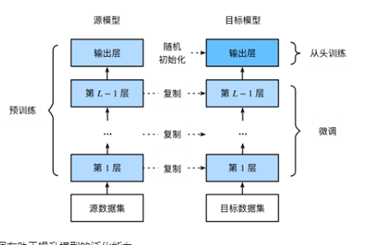2016-12-01 18:01:09 pirage 阅读数 10942
• ###### Python文本数据分析--实战视频教学

购买课程后，可扫码进入学习群，获取唐宇迪老师答疑 课程首先概述文本分析的基本概念,整个课程围绕案例进行：新闻分类任务。案例从零开始讲解如何使用Python库进行分析与建模的工作。案例中实例演示如何从杂乱的文本数据开始进行分词预处理到后应用贝叶斯算法进行分类预测。 专属会员卡更超值：http://edu.csdn.net/lecturer/1079

4147 人正在学习 去看看 唐宇迪

### 分词原理

1. 对语料进行处理，使用word2vec对语料的字进行嵌入，每个字特征为50维。
2. 得到字嵌入后，用字嵌入特征喂给双向LSTM， 对输出的隐层加一个线性层，然后加一个CRF就得到本文实现的模型。s(X,y)=i=0nAyi,yi+1+i=1nPi,yi

p(y|X)=es(X,y)y˘YXes(X,y˘)

log(p(y|X))=s(X,y)log(y˘YXes(X,y˘))

y=argmaxy˘YXs(X,y˘)

### 代码结构与实践

koth大神开源的项目地址为：https://github.com/koth/kcws

• process_anno_file.py 将人民网2014训练语料进行单字切割，包括标点符号
• generate_training.py 生成单字的vector之后，处理每篇训练语料，以“。”划分为句子，对每个句子，如果长度大于MAX_LEN(默认设置为80，在代码里改)，不处理，Long lines加一，如果长度小于MAX_LEN，则生成长度为160的vector，前80为单字在字典中的index，不足80的补0，后80为每个字对应的SBME标记（S表示单字，B表示开始，M表示中间，E表示结尾）。
• filter_sentence.py 将语料切分为训练集和测试集，作者将含有两个字以下的句子过滤掉，剩下的按照二八分，测试集最多8000篇。
• train_cws_lstm.py 主要训练代码。

1. 之前安装tensorflow的时候没有用bazel，不了解bazel的工作方式和原理，但是这个项目必须要用，因为需要用到third_party中word2vec的类。（可以将word2vec的某些类构建为python可以import的类？）
2. 已安装的0.8.0版本的tensorflow没有实现crf，需要升级。
3. 安装tensorflow 0.11.0版本后运行，出现PyUnicodeUCS4_AsUTF8String的错误，查找后发现是当前安装的python默认是unicode=ucs2，需要重新编译安装python。编译的时候设置./configure –enable-unicode=ucs4 。
4. numpy，scipy都需要重新build，setup。

### 主要代码分析

def main(unused_argv):
curdir = os.path.dirname(os.path.realpath(__file__))
trainDataPath = tf.app.flags.FLAGS.train_data_path
if not trainDataPath.startswith("/"):
trainDataPath = curdir + "/" + trainDataPath
graph = tf.Graph()
with graph.as_default():
model = Model(FLAGS.embedding_size, FLAGS.num_tags,
FLAGS.word2vec_path, FLAGS.num_hidden)
print("train data path:", trainDataPath)
# 读取训练集batch大小的feature和label，各为80大小的数组
X, Y = inputs(trainDataPath)
# 读取测试集所有数据的feature和label，各为80大小的数组
# 计算训练集的损失
total_loss = model.loss(X, Y)
train_op = train(total_loss)
# 在测试集上做评测
test_unary_score, test_sequence_length = model.test_unary_score()
# 创建Supervisor管理模型的分布式训练
sv = tf.train.Supervisor(graph=graph, logdir=FLAGS.log_dir)
with sv.managed_session(master='') as sess:
# actual training loop
training_steps = FLAGS.train_steps
for step in range(training_steps):
if sv.should_stop():
break
try:
_, trainsMatrix = sess.run(
[train_op, model.transition_params])
# for debugging and learning purposes, see how the loss gets decremented thru training steps
if step % 100 == 0:
print("[%d] loss: [%r]" % (step, sess.run(total_loss)))
if step % 1000 == 0:
test_evaluate(sess, test_unary_score,
test_sequence_length, trainsMatrix,
model.inp, tX, tY)
except KeyboardInterrupt, e:
sv.saver.save(sess,
FLAGS.log_dir + '/model',
global_step=step + 1)
raise e
sv.saver.save(sess, FLAGS.log_dir + '/finnal-model')
sess.close()

Class Model：

def __init__(self, embeddingSize, distinctTagNum, c2vPath, numHidden):
self.embeddingSize = embeddingSize
self.distinctTagNum = distinctTagNum
self.numHidden = numHidden
self.words = tf.Variable(self.c2v, name="words")
with tf.variable_scope('Softmax') as scope:
self.W = tf.get_variable(
shape=[numHidden * 2, distinctTagNum],
initializer=tf.truncated_normal_initializer(stddev=0.01),
name="weights",
regularizer=tf.contrib.layers.l2_regularizer(0.001))
self.b = tf.Variable(tf.zeros([distinctTagNum], name="bias"))
self.trains_params = None
self.inp = tf.placeholder(tf.int32,
shape=[None, FLAGS.max_sentence_len],
name="input_placeholder")
pass

def length(self, data):
used = tf.sign(tf.reduce_max(tf.abs(data), reduction_indices=2))
length = tf.reduce_sum(used, reduction_indices=1)
length = tf.cast(length, tf.int32)
return length

def inference(self, X, reuse=None, trainMode=True):
word_vectors = tf.nn.embedding_lookup(self.words, X) # 按照X顺序返回self.words中的第X行，返回的结果组成tensor。
length = self.length(word_vectors)
# length是shape为[batch_size]大小值为句子长度的vector
length_64 = tf.cast(length, tf.int64)
if trainMode:  # 训练的时候启用dropout，测试的时候关键dropout
word_vectors = tf.nn.dropout(word_vectors, 0.5)  # 将word_vectors按照50%的概率丢弃某些词，tf增加的一个处理是将其余的词scale 1/0.5
with tf.variable_scope("rnn_fwbw", reuse=reuse) as scope:
forward_output, _ = tf.nn.dynamic_rnn(
tf.nn.rnn_cell.LSTMCell(self.numHidden),
word_vectors,
dtype=tf.float32,
sequence_length=length,
scope="RNN_forward")
backward_output_, _ = tf.nn.dynamic_rnn(
tf.nn.rnn_cell.LSTMCell(self.numHidden),
inputs=tf.reverse_sequence(word_vectors,
length_64,
seq_dim=1),
# 训练和测试的时候，inputs的格式不同。训练时，tensor shape是[batch_size, max_time,input_size]
# 测试时，tensor shape是[max_time,batch_size,input_size].
# tf.reverse_sequence作用就是指定在列上操作（batch_dim表示按行操作）
dtype=tf.float32,
sequence_length=length,
scope="RNN_backword")
# tf.nn.dynamic_rnn(cell, inputs, sequence_length,time_major,...)主要参数：
# cell：搭建好的网络，这里用LSTMCell(num_cell)，num_cell表示一个lstm单元输出的维数（100）
# inputs：word_vectors，它的shape由time_major决定，默认是false，即[batch_size，max_time，input_size]，如果是测试
#           过程，time_major设置为True，shape为[max_time，batch_size，input_size]，这里直接做了reverse，省去了time_major设置。
#         其中，batch_size=100, max_time=80句子最大长度，input_size字的向量的长度。
# sequence_length：shape[batch_size]大小的值为句子最大长度的tensor。
# 输出：
#   outputs：[batch_size, max_time, cell.output_size]
#   state: shape取决于LSTMCell中state_size的设置，返回Tensor或者tuple。

backward_output = tf.reverse_sequence(backward_output_,
length_64,
seq_dim=1)
# 这里的reverse_sequence同上。
output = tf.concat(2, [forward_output, backward_output])
# 连接两个三维tensor，2表示按照列连接（0表示纵向，1表示行）
# 连接后，output的shape:[batch_size, max_time, 2*cell.output_size],即[100， 80， 2*50]
output = tf.reshape(output, [-1, self.numHidden * 2])
# reshape后，output的shape:[batch_size, self.numHidden * 2],即[100, 200]
matricized_unary_scores = tf.batch_matmul(output, self.W)
# 得到未归一化的CRF输出
# 点乘W的shape[ 100*2, 4],生成[batch_size, 4]大小的matricized_unary_scores
unary_scores = tf.reshape(
matricized_unary_scores,
[-1, FLAGS.max_sentence_len, self.distinctTagNum])
# reshape后，unary_scores大小为[batch_size，80， 4]
return unary_scores, length

def loss(self, X, Y):
P, sequence_length = self.inference(X)
# CRF损失计算，训练的时候使用，测试的时候用viterbi解码
log_likelihood, self.transition_params = tf.contrib.crf.crf_log_likelihood(
P, Y, sequence_length)
# crf_log_likelihood参数(inputs,tag_indices, sequence_lengths)
#   inputs:大小为[100, 80, 4]的tensor，CRF层的输入
#   tag_indices:大小为[100, 80]的矩阵
#   sequence_length：大小 值为80的向量。
# 输出：
#   log_likelihood：[batch_size]大小的vector，log-likelihood值
#   transition_params：[4,4]大小的矩阵
loss = tf.reduce_mean(-log_likelihood)
return loss

fp = open(path, "r")
ss = line.split(" ")
total = int(ss)
dim = int(ss)
assert (dim == (FLAGS.embedding_size))
ws = []
mv = [0 for i in range(dim)]
# The first for 0
ws.append([0 for i in range(dim)])
for t in range(total):
ss = line.split(" ")
assert (len(ss) == (dim + 1))
vals = []
for i in range(1, dim + 1):
fv = float(ss[i])
mv[i - 1] += fv
vals.append(fv)
ws.append(vals)
for i in range(dim):
mv[i] = mv[i] / total
ws.append(mv)
fp.close()
return np.asarray(ws, dtype=np.float32)

def test_unary_score(self):
P, sequence_length = self.inference(self.inp,
reuse=True,
trainMode=False)
return P, sequence_length

### 总结

2019-09-10 17:20:31 isxixi 阅读数 209
• ###### Python文本数据分析--实战视频教学

购买课程后，可扫码进入学习群，获取唐宇迪老师答疑 课程首先概述文本分析的基本概念,整个课程围绕案例进行：新闻分类任务。案例从零开始讲解如何使用Python库进行分析与建模的工作。案例中实例演示如何从杂乱的文本数据开始进行分词预处理到后应用贝叶斯算法进行分类预测。 专属会员卡更超值：http://edu.csdn.net/lecturer/1079

4147 人正在学习 去看看 唐宇迪

1. 基于字典的分词方法
2. 基础规则的分词方法
3. 基于统计的分词方法（统计在一段话中出现频率最的那个分词字段）
4. 基于深度学的的方法（通过大量的数据的学习）

1. one-hot
2. word2vec 将词映射到多维空间里

1. RNN（循环神经网络）
特点：记忆是短期，梯度消失以及梯度爆炸‘’
2. LSTM（长短期记忆网络） 是 RNN 的进一步优化
特点：克服 RNN 梯度消失的问题，而且能学习到长距离的信息
3. BILSTM （双向长短期神经网络）

CRF：条件随机场是标记、分割结构化数据的统计模型。CRF 优于隐马尔可夫模型在于放松了 HMM 所需的独立性假设。另外避免了标签偏差问题。CRF 训练的损失函数是凸函数，全局收敛，具有非常好的实用性。

jieba 是结合基于规则和基于统计的分词工具。
jieba 有三种分词模式，分别是精确模式，全模式和搜索引擎模式

1. 输入层
使用one-hot编码。
2. 隐藏层
隐藏层的神经单元数量代表着每一个词用向量表示的维度。
3. 输出层
七、分词的评估标准
精确率：正确的分词个数/总分词的个数
召回率：正确的个数/标准分词的个数
F值：正确率召回率2/(正确率+召回率)
错误率:错误分词的个数/标准分词的个数

2019-08-27 09:53:03 emdsh 阅读数 121
• ###### Python文本数据分析--实战视频教学

购买课程后，可扫码进入学习群，获取唐宇迪老师答疑 课程首先概述文本分析的基本概念,整个课程围绕案例进行：新闻分类任务。案例从零开始讲解如何使用Python库进行分析与建模的工作。案例中实例演示如何从杂乱的文本数据开始进行分词预处理到后应用贝叶斯算法进行分类预测。 专属会员卡更超值：http://edu.csdn.net/lecturer/1079

4147 人正在学习 去看看 唐宇迪

one-hot将每个单词与一个唯一的整数索引相关联，将整数索引i转换为长度为N的二进制向量。One-hot编码是标记转化为向量最基本最常用的方法，得到的是二进制的，稀疏的维度很高的向量。

使用预训练的词嵌入：原理同图像分类中使用的预训练的卷积神经网络一样，这种词嵌入利用词频统计计算得出。

处理IMDB数据的原始文本
import os
imdb_dir='D:\\jupyter_code\\GloVe\\aclImdb'
train_dir=os.path.join(imdb_dir,'train')

labels=[]
texts=[]

for label_type in ['neg','pos']:
dir_name=os.path.join(train_dir,label_type)
for fname in os.listdir(dir_name):
if fname[-4:]=='.txt':
f=open(os.path.join(dir_name,fname),encoding='UTF-8')
f.close()
if label_type=='neg':
labels.append(0)
else:
labels.append(1)

#对imdb原始数据的文本进行分词
from keras.preprocessing.text import Tokenizer
import numpy as np
#在100个单词后截断评论
maxlen=100
#200个样本上训练
training_samples=200
#在10000个样本上验证
validation_samples=10000
#只考虑前10000个最常见的单词
max_words=10000

tokenizer=Tokenizer(num_words=max_words)
#根据文本列表更新词汇表
tokenizer.fit_on_texts(texts)
sequences=tokenizer.texts_to_sequences(texts)

word_index=tokenizer.word_index
print('Found %s unique tokens.'%len(word_index))

labels=np.asarray(labels)
print(data.shape)
print(labels.shape)

#打乱数据在划分数据
indices=np.arange(data.shape)
np.random.shuffle(indices)
data=data[indices]
labels=labels[indices]

x_train=data[:training_samples]
y_train=labels[:training_samples]
x_val=data[training_samples:training_samples+validation_samples]
y_val=labels[training_samples:training_samples+validation_samples]

#解析GloVe嵌入文件
glove_dir='D:\\jupyter_code\\GloVe\\glove.6B'

embeddings_index={}
f=open(os.path.join(glove_dir,'glove.6B.100d.txt'),encoding='UTF-8')
for line in f:
values=line.split()
word=values
coefs=np.asarray(values[1:],dtype='float32')
embeddings_index[word]=coefs
f.close()

print('Found %s word vectors'%len(embeddings_index))

#准备GloVe词嵌入词库
embedding_dim=100
embedding_matrix=np.zeros((max_words,embedding_dim))
for word, i in word_index.items():
if i<max_words:
embedding_vector=embeddings_index.get(word)
if embedding_vector is not None:
embedding_matrix[i]=embedding_vector

#定义模型
from keras.models import Sequential
from keras.layers import Embedding,Flatten,Dense

model=Sequential()
model.summary()

#预训练的词嵌入加载到Embedding层，冻结Embedding
model.layers.set_weights([embedding_matrix])
model.layers.trainable=False

#模型训练与评估
model.compile(optimizer='rmsprop',loss='binary_crossentropy',metrics=['acc'])
history=model.fit(x_train,y_train,epochs=10,batch_size=32,validation_data=(x_val,y_val))
model.save_weights('pre_trained_glove_model.h5')

#在不使用预训练词嵌入的情况下，训练相同的模型
from keras.models import Sequential
from keras.layers import Embedding,Flatten,Dense

model=Sequential()
model.summary()

model.compile(optimizer='rmsprop',loss='binary_crossentropy',metrics=['acc'])
history=model.fit(x_train,y_train,epochs=10,batch_size=32,validation_data=(x_val,y_val))

#对测试集数据进行分词
test_dir=os.path.join(imdb_dir,'test')
labels=[]
texts=[]

for label_type in ['neg','pos']:
dir_name=os.path.join(test_dir,label_type)
for fname in sorted(os.listdir(dir_name)):
if fname[-4:]=='.txt':
f=open(os.path.join(dir_name,fname),encoding='UTF-8')
f.close()
if label_type=='neg':
labels.append(0)
else:
labels.append(1)

sequences=tokenizer.texts_to_sequences(texts)
y_test=np.asarray(labels)

model.evaluate(x_test,y_test)

2020-02-14 17:51:44 qq_30205387 阅读数 30
• ###### Python文本数据分析--实战视频教学

购买课程后，可扫码进入学习群，获取唐宇迪老师答疑 课程首先概述文本分析的基本概念,整个课程围绕案例进行：新闻分类任务。案例从零开始讲解如何使用Python库进行分析与建模的工作。案例中实例演示如何从杂乱的文本数据开始进行分词预处理到后应用贝叶斯算法进行分类预测。 专属会员卡更超值：http://edu.csdn.net/lecturer/1079

4147 人正在学习 去看看 唐宇迪

Softmax与分类模型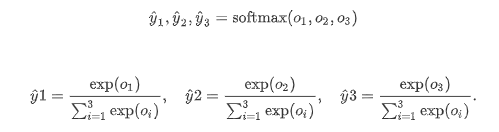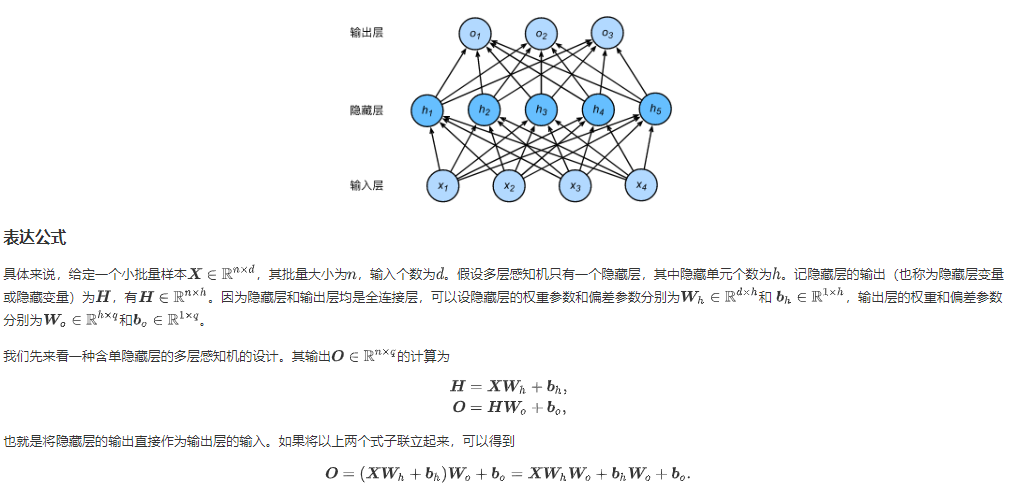1. 读入文本
2. 分词
3. 建立字典，将每个词映射到一个唯一的索引（index）
4. 将文本从词的序列转换为索引的序列，方便输入模型

lines = [re.sub(’[^a-z]+’, ’ ', line.strip().lower()) for line in f]
%将文本中的大写替换成小写，并将由非英文字母构成的子串替换成空格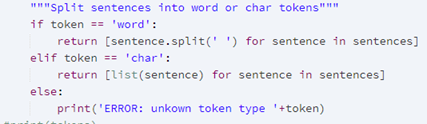for idx, token in enumerate(self.idx_to_token)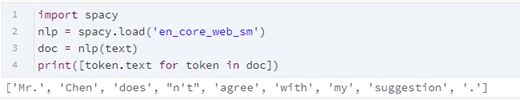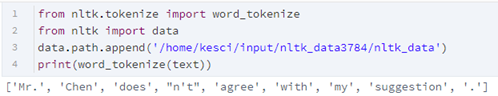## 语言模型

N元语法的缺陷： 参数空间过大（v+v2+v3+…），数据稀疏（齐夫定律：大多数的单词的词频低）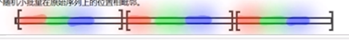## 循环神经网络基础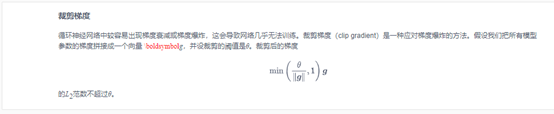## 过拟合欠拟合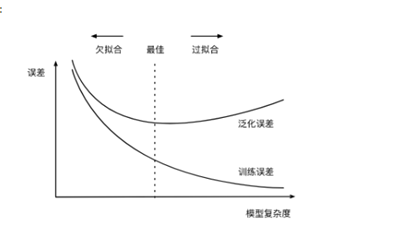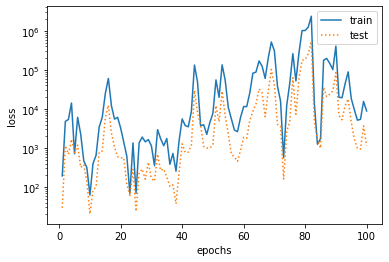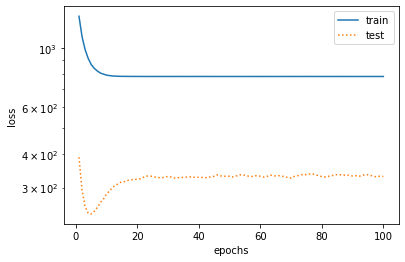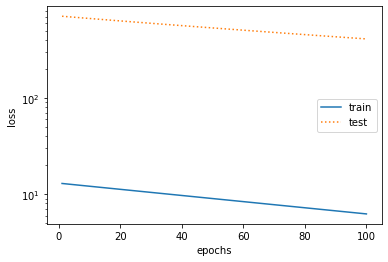1. 获得更多的训练样本。比如通过数据增强方式来扩充或者GAN来合成。
2. 降低模型的复杂度。
3. 正则化
4. 集成学习方法
5. dropout

6. 添加新的特征
7. 增加模型的复杂度，加强模型的拟合能力
8. 减小正则化系数

## 机器翻译及相关技术

Encoder和decoder框架
Sequence to Sequence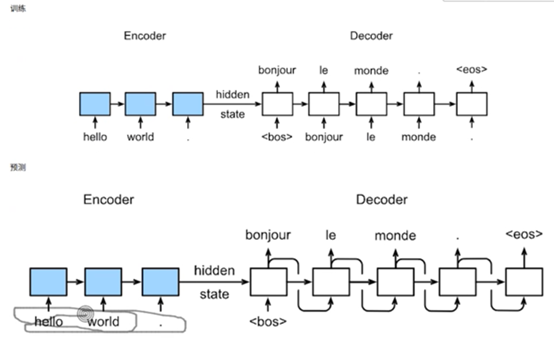## 注意力机制与seq2seq模型

Transform模型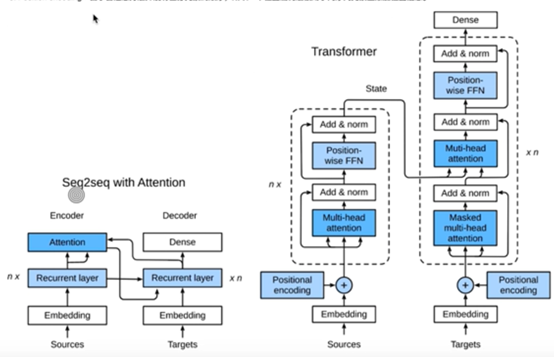## 卷积神经网络进阶

NiN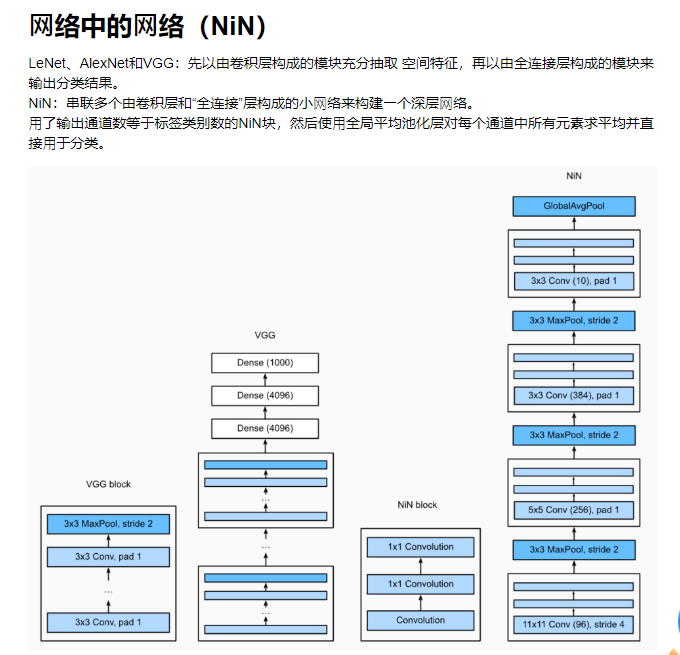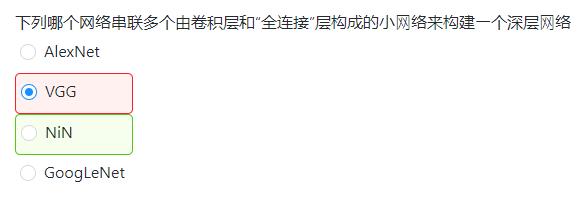https://www.zhihu.com/question/49230360

BN处理

## 凸优化

• 优化方法目标：训练集损失函数值
• 深度学习目标：测试集损失函数值（泛化性）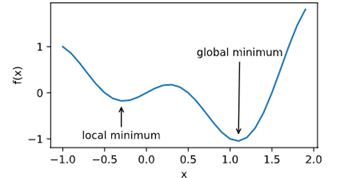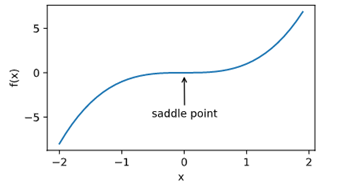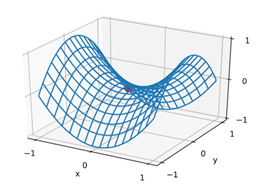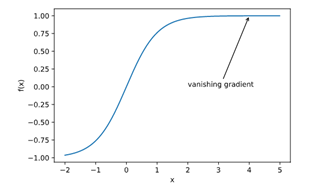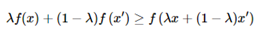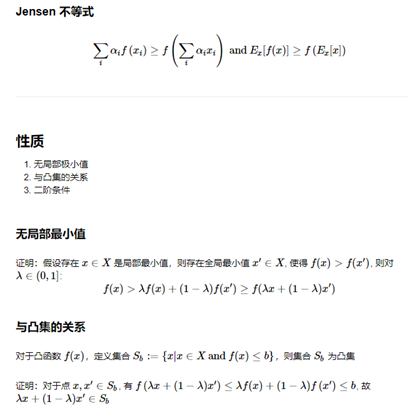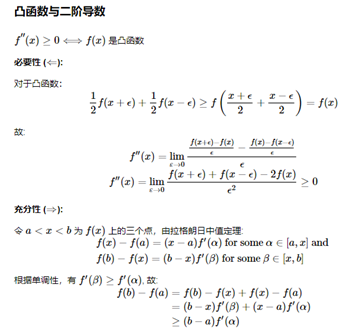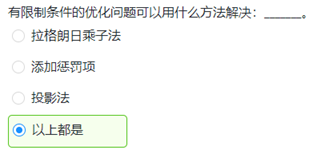## 梯度下降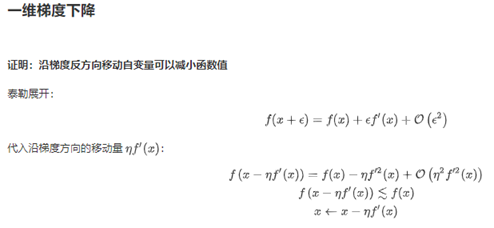（在一定程度上依赖于学习率）梯度下降的自适应方法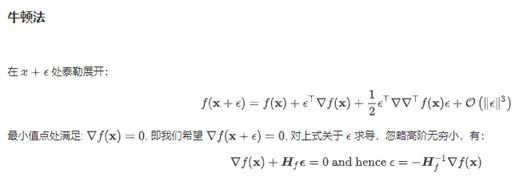## 图像风格迁移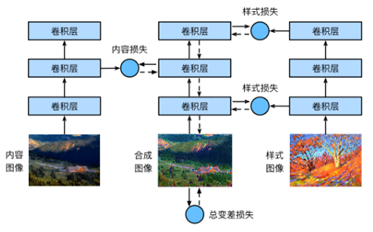## 数据增强

Pytorch中的torchvision.transforms模块用于数据增强
RandomHorizontalFlip实例来实现一半概率的图像水平（左右）翻转
RandomVerticalFlip实例来实现一半概率的图像垂直（上下）翻转
RandomResizedCrop随机裁剪
ColorJitter变化颜色

## 模型微调